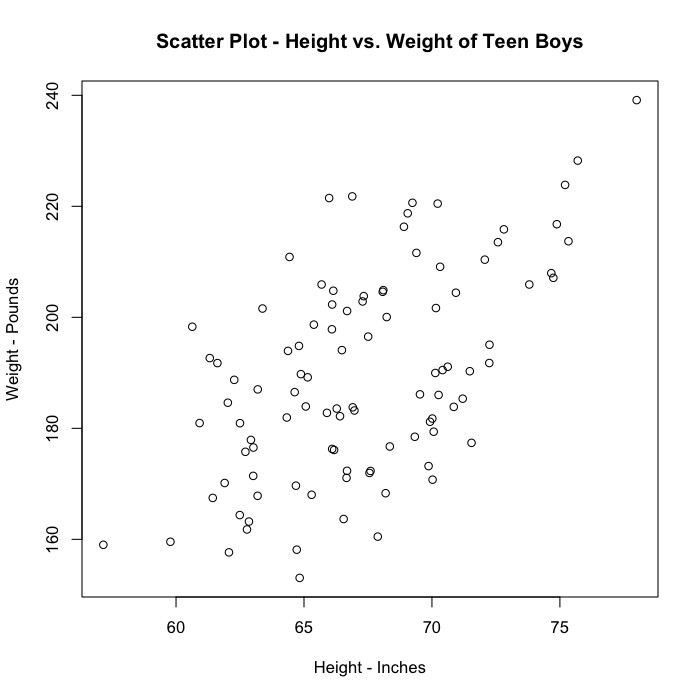# Scatter PlotThe scatter plot is a point plot in which each point represents two variables, one on the x-axis and one on the y-axis, describing the same object. For example, this hypothetical scatter plot shows the heights and weights of 100 teen-aged boys. Each point represents one boy, with the x-axis showing his height and the y-axis showing his weight.

The scatter plot is useful for determining whether or not there is an apparent relationship or correlation between two variables. The more the points cluster close to a diagonal line drawn through the “center of all the points”, the stronger the apparent relationship between the two variables. The more the points resemble an amorphous cloud, the weaker the apparent relationship. The slope of the line reflects the positive or negative nature of the correlation.

In this case, the data suggests that the taller boys tend also to be heavier.